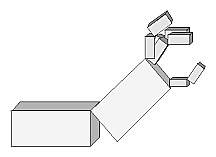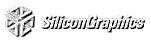# Chapter 3 Viewing

Chapter Objectives

After reading this chapter, you'll be able to do the following:

View a geometric model in any orientation by transforming it in three-dimensional space

Control the location in three-dimensional space from which the model is viewed

Clip undesired portions of the model out of the scene that's to be viewed

Manipulate the appropriate matrix stacks that control model transformation for viewing and project the model onto the screen

Combine multiple transformations to mimic sophisticated systems in motion, such as a solar system or an articulated robot arm

Chapter 2 explained how to instruct OpenGL to draw the geometric models you want displayed in your scene. Now you must decide how you want to position the models in the scene, and you must choose a vantage point from which to view the scene. You can use the default positioning and vantage point, but most likely you want to specify them.

Look at the image on the cover of this book. The program that produced that image contained a single geometric description of a building block. Each block was carefully positioned in the scene: Some blocks were scattered on the floor, some were stacked on top of each other on the table, and some were assembled to make the globe. Also, a particular viewpoint had to be chosen. Obviously, we wanted to look at the corner of the room containing the globe. But how far away from the scene - and where exactly - should the viewer be? We wanted to make sure that the final image of the scene contained a good view out the window, that a portion of the floor was visible, and that all the objects in the scene were not only visible but presented in an interesting arrangement. This chapter explains how to use OpenGL to accomplish these tasks: how to position and orient models in three-dimensional space and how to establish the location - also in three-dimensional space - of the viewpoint. All of these factors help determine exactly what image appears on the sceen.

You want to remember that the point of computer graphics is to create a two-dimensional image of three-dimensional objects (it has to be two-dimensional because it's drawn on the screen), but you need to think in three-dimensional coordinates while making many of the decisions that determine what gets drawn on the screen. A common mistake people make when creating three-dimensional graphics is to start thinking too soon that the final image appears on a flat, two-dimensional screen. Avoid thinking about which pixels need to be drawn, and instead try to visualize three-dimensional space. Create your models in some three-dimensional universe that lies deep inside your computer, and let the computer do its job of calculating which pixels to color.

A series of three computer operations convert an object's three-dimensional coordinates to pixel positions on the screen:

Transformations, which are represented by matrix multiplication, include modeling, viewing, and projection operations. Such operations include rotation, translation, scaling, reflecting, orthographic projection, and perspective projection. Generally, you use a combination of several transformations to draw a scene.

Since the scene is rendered on a rectangular window, objects (or parts of objects) that lie outside the window must be clipped. In three-dimensional computer graphics, clipping occurs by throwing out objects on one side of a clipping plane.

Finally, a correspondence must be established between the transformed coordinates and screen pixels. This is known as a viewport transformation.

This chapter describes all of these operations, and how to control them, in the following major sections:
"Overview: The Camera Analogy" gives an overview of the transformation process by describing the analogy of taking a photograph with a camera, presents a simple example program that transforms an object, and briefly describes the basic OpenGL transformation commands.

"Viewing and Modeling Transformations" explains in detail how to specify and to imagine the effect of viewing and modeling transformations. These transformations orient the model and the camera relative to each other to obtain the desired final image.

"Projection Transformations" describes how to specify the shape and orientation of the viewing volume. The viewing volume determines how a scene is projected onto the screen (with a perspective or orthographic projection) and which objects or parts of objects are clipped out of the scene.

"Viewport Transformation" explains how to control the conversion of three-dimensional model coordinates to screen coordinates.

"Troubleshooting Transformations" presents some tips for discovering why you might not be getting the desired effect from your modeling, viewing, projection, and viewport transformations.

"Manipulating the Matrix Stacks" discusses how to save and restore certain transformations. This is particularly useful when you're drawing complicated objects that are built up from simpler ones.

"Additional Clipping Planes" describes how to specify additional clipping planes beyond those defined by the viewing volume.

"Examples of Composing Several Transformations" walks you through a couple of more complicated uses for transformations.

## Overview: The Camera Analogy

The transformation process to produce the desired scene for viewing is analogous to taking a photograph with a camera. As shown in Figure 3-1 , the steps with a camera (or a computer) might be the following:
Setting up your tripod and pointing the camera at the scene (viewing transformation).

Arranging the scene to be photographed into the desired composition (modeling transformation).

Choosing a camera lens or adjusting the zoom (projection transformation).

Determining how large you want the final photograph to be - for example, you might want it enlarged (viewport transformation).

After these steps are performed, the picture can be snapped, or the scene can be drawn.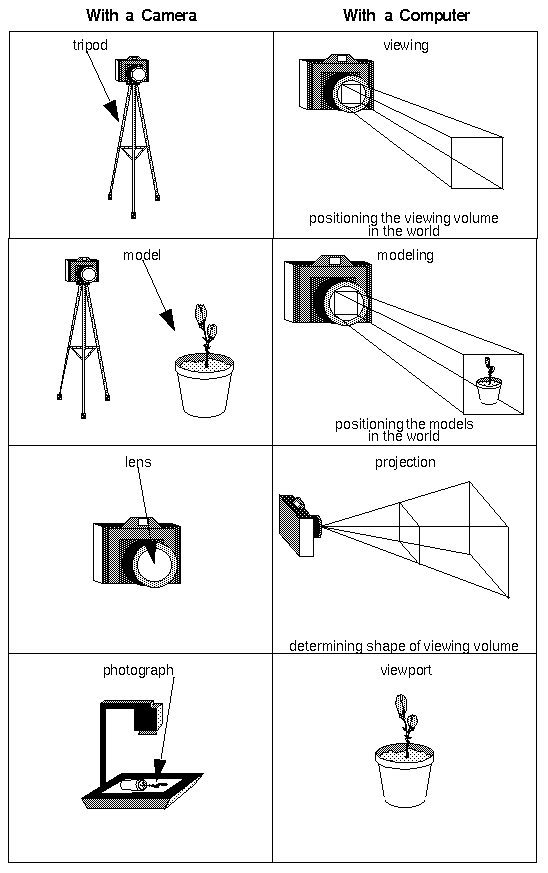Figure 3-1 : The Camera Analogy

Note that these steps correspond to the order in which you specify the desired transformations in your program, not necessarily the order in which the relevant mathematical operations are performed on an object's vertices. The viewing transformations must precede the modeling transformations in your code, but you can specify the projection and viewport transformations at any point before drawing occurs. Figure 3-2 shows the order in which these operations occur on your computer.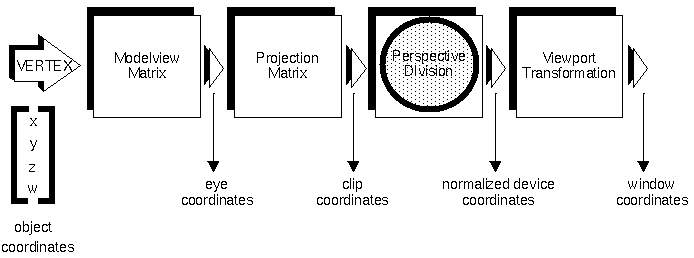Figure 3-2 : Stages of Vertex Transformation

To specify viewing, modeling, and projection transformations, you construct a 4 × 4 matrix M, which is then multiplied by the coordinates of each vertex v in the scene to accomplish the transformation

v'=Mv

(Remember that vertices always have four coordinates (x ,y, z, w), though in most cases w is 1 and for two-dimensional data z is 0.) Note that viewing and modeling transformations are automatically applied to surface normal vectors, in addition to vertices. (Normal vectors are used only in eye coordinates.) This ensures that the normal vector's relationship to the vertex data is properly preserved.

The viewing and modeling transformations you specify are combined to form the modelview matrix, which is applied to the incoming object coordinates to yield eye coordinates. Next, if you've specified arbitrary clipping planes to remove certain objects from the scene or to provide cutaway views of objects, these clipping planes are applied.

After that, OpenGL applies the projection matrix to yield clip coordinates. This transformation defines a viewing volume; objects outside this volume are clipped so that they're not drawn in the final scene. After this point, the perspective division is performed by dividing coordinate values by w, to produce normalized device coordinates. (See Appendix G for more information about the meaning of the w coordinate and how it affects matrix transformations.) Finally, the transformed coordinates are converted to window coordinates by applying the viewport transformation. You can manipulate the dimensions of the viewport to cause the final image to be enlarged, shrunk, or stretched.

You might correctly suppose that the x and y coordinates are sufficient to determine which pixels need to be drawn on the screen. However, all the transformations are performed on the z coordinates as well. This way, at the end of this transformation process, the z values correctly reflect the depth of a given vertex (measured in distance away from the screen). One use for this depth value is to eliminate unnecessary drawing. For example, suppose two vertices have the same x and y values but different z values. OpenGL can use this information to determine which surfaces are obscured by other surfaces and can then avoid drawing the hidden surfaces. (See Chapter 10 for more information about this technique, which is called hidden-surface removal.)

As you've probably guessed by now, you need to know a few things about matrix mathematics to get the most out of this chapter. If you want to brush up on your knowledge in this area, you might consult a textbook on linear algebra.

### A Simple Example: Drawing a Cube

Example 3-1 draws a cube that's scaled by a modeling transformation (see Figure 3-3 ). The viewing transformation used is a simple translation down the z-axis. A projection transformation and a viewport transformation are also specified. The rest of this section walks you through Example 3-1 and briefly explains the transformation commands it uses. The succeeding sections contain the complete, detailed discussion of all OpenGL's transformation commands.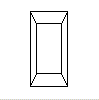Figure 3-3 : A Transformed Cube

Example 3-1 : A Transformed Cube: cube.c

```#include <GL/gl.h>
#include <GL/glu.h>
#include "aux.h"

void display (void)
{
glClear(GL_COLOR_BUFFER_BIT);
glColor3f (1.0, 1.0, 1.0);
glLoadIdentity ();             /* clear the matrix */
glTranslatef (0.0, 0.0, -5.0); /* viewing transformation */
glScalef (1.0, 2.0, 1.0);      /* modeling transformation */
auxWireCube(1.0);    /*  draw the cube */
glFlush();
}

void myinit (void)
{
}

void myReshape(GLsizei w, GLsizei h)
{
glMatrixMode (GL_PROJECTION);    /* prepare for and then */
glLoadIdentity ();               /* define the projection */
glFrustum (-1.0, 1.0, -1.0, 1.0, /* transformation */
1.5, 20.0);
glMatrixMode (GL_MODELVIEW);  /* back to modelview matrix */
glViewport (0, 0, w, h);      /* define the viewport */
}

int main(int argc, char** argv)
{
auxInitDisplayMode (AUX_SINGLE | AUX_RGBA);
auxInitPosition (0, 0, 500, 500);
auxInitWindow (argv);
myinit ();
auxReshapeFunc (myReshape);
auxMainLoop(display);
}```

#### The Viewing Transformation

Recall that the viewing transformation is analogous to positioning and aiming a camera. In this code example, before the viewing transformation can be specified, the current matrix is set to the identity matrix with glLoadIdentity(). This step is necessary since most of the transformation commands multiply the current matrix by the specified matrix and then set the result to be the current matrix. If you don't clear the current matrix by loading it with the identity matrix, you continue to combine previous transformation matrices with the new one you supply. In some cases, you do want to perform such combinations, but you also need to clear the matrix sometimes.

Once the matrix is initialized, the viewing transformation is specified with glTranslatef(). The arguments for this command indicate how the camera should be translated (moved) in the x, y, and z directions. The arguments used here move the camera 5 units in the negative z direction. By default, the camera as well as any objects in the scene are originally situated at the origin; also, the camera initially points down the negative z-axis. Thus, the particular viewing transformation used here has the effect of pulling the camera away from where the cube is, but it leaves the camera pointing at the object. If the camera needed to be pointed in another direction, you could have used the glRotatef() command to change its orientation. Viewing transformations are discussed in detail in "Viewing and Modeling Transformations."

#### The Modeling Transformation

You use the modeling transformation to position and orient the model. For example, you can rotate, translate, or scale the model - or perform some combination of these operations. Rotating and translating are performed using the commands already mentioned - glRotatef() and glTranslatef(). In this example, however, the modeling transformation is invoked with glScalef(). The arguments for this command specify how scaling should occur along the three axes. If all the arguments are 1.0, this command has no effect; in Example 3-1 , the cube is drawn twice as large in the y direction. Thus, if one corner of the cube had originally been at (3.0, 3.0, 3.0), that corner would wind up being drawn at (3.0, 6.0, 3.0). The effect of this modeling transformation is to transform the cube so that it isn't a cube but a rectangular box.

Note that instead of pulling the camera back away from the cube (with a viewing transformation) so that it could be viewed, you could have moved the cube away from the camera (with a modeling transformation). This duality in the nature of viewing and modeling transformations is why you need to think about the effect of both types of transformations simultaneously. It doesn't make sense to try to separate the effects, but sometimes it's easier to think about them one way rather than the other. This is also why modeling and viewing transformations are combined into the modelview matrix before the transformations are applied. "Viewing and Modeling Transformations," explains in more detail how to think about modeling and viewing transformations and how to specify them so that you get the result you want.

Also note that the modeling and viewing transformations are included in the display() routine, along with the call that's used to draw the cube, auxWireCube(). This way, display() can be used repeatedly to draw the contents of the window if, for example, the window is moved or uncovered, and you've ensured that each time, the cube is drawn in the desired way, with the appropriate transformations. The potential repeated use of display() underscores the need to load the identity matrix before performing the viewing and modeling transformations, especially when other transformations might be performed between calls to display().

#### The Projection Transformation

Specifying the projection transformation is like choosing a lens for a camera. You can think of this transformation as determining what the field of view or viewing volume is and therefore what objects are inside it and to some extent how they look. This is equivalent to choosing among wide-angle, normal, and telephoto lenses, for example. With a wide-angle lens, you can include a wider scene in the final photograph than with a telephoto lens, but a telephoto lens allows you to photograph objects as though they're closer to you than they actually are. In computer graphics, you don't have to pay \$10,000 for a 2000-millimeter telephoto lens; once you've bought your graphics workstation, all you need to do is use a smaller number for your field of view.

In addition to the field-of-view considerations, the projection transformation determines how objects are projected onto the screen, as its name suggests. Two basic types of projections are provided for you by OpenGL, along with several corresponding commands for describing the relevant parameters in different ways. One type is the perspective projection, which matches how you see things in daily life. Perspective makes objects that are farther away appear smaller; for example, it makes railroad tracks appear to converge in the distance. If you're trying to make realistic pictures, you'll want to choose perspective projection, which is specified with the glFrustum() command in this code example.

The other type of projection is orthographic, which maps objects directly onto the screen without affecting their relative size. Orthographic projection is used in architectural and computer-aided design applications where the final image needs to reflect the measurements of objects rather than how they might look. Architects create perspective drawings to show how particular buildings or interior spaces look when viewed from various vantage points; the need for orthographic projection arises when blueprint plans or elevations are generated, which are used in the construction of buildings. "Projection Transformations," discusses the ways to specify both kinds of projection transformations in more detail.

Before glFrustum() can be called to set the projection transformation, some preparation needs to happen. As shown in the myReshape() routine in Example 3-1 , the command called glMatrixMode() is used first, with the argument GL_PROJECTION. This indicates that the current matrix specifies the projection transformation; the following transformation calls then affect the projection matrix. As you can see, a few lines later glMatrixMode() is called again, this time with GL_MODELVIEW as the argument. This indicates that succeeding transformations now affect the modelview matrix instead of the projection matrix. See "Manipulating the Matrix Stacks," for more information about how to control the projection and modelview matrices.

Note that glLoadIdentity() is used to initialize the current projection matrix so that only the specified projection transformation has an effect. Now glFrustum() can be called, with arguments that define the parameters of the projection transformation. In this example, both the projection transformation and the viewport transformation are contained in the myReshape() routine, which is called when the window is first created and whenever the window is moved or reshaped. This makes sense, since both projecting and applying the viewport relate directly to the screen, and specifically to the size of the window on the screen.

#### The Viewport Transformation

Together, the projection transformation and the viewport transformation determine how a scene gets mapped onto the computer screen. The projection transformation specifies the mechanics of how the mapping should occur, and the viewport indicates the shape of the available screen area into which the scene is mapped. Since the viewport specifies the region the image occupies on the computer screen, you can think of the viewport transformation as defining the size and location of the final processed photograph - whether it should be enlarged or shrunk, for example.

The arguments to glViewport() describe the origin of the available screen space within the window - (0, 0) in this example - and the width and height of the available screen area, all measured in pixels on the screen. This is why this command needs to be called within myReshape() - if the window changes size, the viewport needs to change accordingly. Note that the width and height are specified using the actual width and height of the window; often, you want to specify the viewport this way rather than giving an absolute size. See "Viewport Transformation," for more information about how to define the viewport.

#### Drawing the Scene

Once all the necessary transformations have been specified, you can draw the scene (that is, take the photograph). As the scene is drawn, OpenGL transforms each vertex of every object in the scene by the modeling and viewing transformations. Each vertex is then transformed as specified by the projection transformation and clipped if it lies outside the viewing volume described by the projection transformation. Finally, the remaining transformed vertices are divided by w and mapped onto the viewport.

### General-Purpose Transformation Commands

This section discusses some OpenGL commands that you might find useful as you specify desired transformations. You've already seen a couple of these commands, glMatrixMode() and glLoadIdentity(). The other two commands described here - glLoadMatrix*() and glMultMatrix*() - allow you to specify any transformation matrix directly and then to multiply the current matrix by that specified matrix. More specific transformation commands - such as glTranslate*() and glScale*() - are described in later sections.

As described in the preceding section, you need to state whether you want to modify the modelview or projection matrix before supplying a transformation command. You do this with glMatrixMode(). When you use nested sets of OpenGL commands that might be called repeatedly, remember to reset the matrix mode correctly. (The glMatrixMode() command can also be used to indicate the texture matrix; texturing is discussed in detail in Chapter 9 .)void glMatrixMode(GLenum mode);

Specifies whether the modelview, projection, or texture matrix will be modified, using the argument GL_MODELVIEW, GL_PROJECTION, or GL_TEXTURE for mode. Subsequent transformation commands affect the specified matrix. Note that only one matrix can be modified at a time. By default, the modelview matrix is the one that's modifiable, and all three matrices contain the identity matrix.

You use the glLoadIdentity() command to clear the currently modifiable matrix for future transformation commands, since these commands modify the current matrix. Typically, you always call this command before specifying projection or viewing transformations, but you might also call it before specifying a modeling transformation.void glLoadIdentity(void);

Sets the currently modifiable matrix to the 4 × 4 identity matrix.

If you want to explicitly specify a particular matrix to be loaded as the current matrix, use glLoadMatrix*(). Similarly, use glMultMatrix*() to multiply the current matrix by the matrix passed in as an argument. The argument for both these commands is a vector of sixteen values (m1, m2, ... , m16) that specifies a matrix M as follows: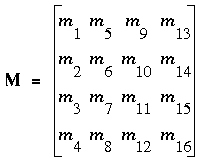Remember that you might be able to maximize efficiency by using display lists to store frequently used matrices (and their inverses) rather than recomputing them; see "Display-List Design Philosophy." (OpenGL implementations often must compute the inverse of the modelview matrix so that normals and clipping planes can be correctly transformed to eye coordinates.)

If you're programming in C, and you declare a matrix as m, then the element m[i][j] is in the ith column and jth row of the OpenGL transformation matrix. This is the reverse of the standard C convention in which m[i][j] is in row i and column j. To avoid confusion, you should declare your matrices as m. void glLoadMatrix{fd}(const TYPE *m);

Sets the sixteen values of the current matrix to those specified by m.

void glMultMatrix{fd}(const TYPE *m);

Multiplies the matrix specified by the sixteen values pointed to by m by the current matrix and stores the result as the current matrix.

All matrix multiplication with OpenGL occurs as follows: Suppose the current matrix is C and the matrix specified with glMultMatrix*() or any of the transformation commands is M. After multiplication, the final matrix is always CM. Since matrix multiplication isn't generally commutative, the order makes a difference.

## Viewing and Modeling Transformations

As noted in "A Simple Example: Drawing a Cube," viewing and modeling transformations are inextricably related in OpenGL and are in fact combined into a single modelview matrix. One of the toughest problems newcomers to computer graphics face is understanding the effects of combined three-dimensional transformations. As you've already seen, there are alternative ways to think about transformations - do you want to move the camera in one direction, or move the object in the opposite direction? Each way of thinking about transformations has advantages and disadvantages, but in some cases one way more naturally matches the effect of the intended transformation. If you can find a natural approach for your particular application, it's easier to visualize the necessary transformations and then write the corresponding code to specify the matrix manipulations. The first part of this section discusses how to think about transformations; later, specific commands are presented. For now, we use only the matrix-manipulation commands you've already seen. Finally, keep in mind that you must call glMatrixMode() with GL_MODELVIEW as its argument prior to performing modeling or viewing transformations.

Let's start with a simple case of two transformations: a 45-degree counterclockwise rotation about the origin around the z-axis, and a translation down the x-axis. Suppose that the object you're drawing is small compared to the translation (so that you can see the effect of the translation), and that it's originally located at the origin. If you rotate the object first and then translate it, the rotated object appears on the x-axis. If you translate it down the x-axis first, however, and then rotate about the origin, the object is on the line y=x, as shown in Figure 3-4 . In general, the order of transformations is critical. If you do transformation A and then transformation B, you almost certainly get something different than if you do them in the opposite order.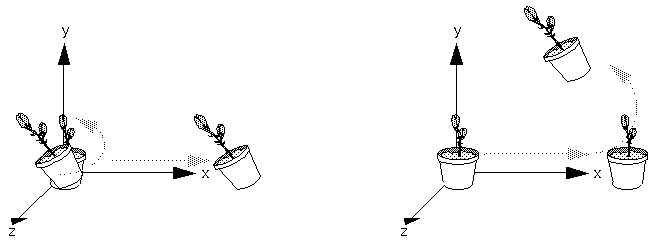Figure 3-4 : Rotating First or Translating First

Now let's talk about the order in which you specify a series of transformations. All viewing and modeling transformations are represented as 4 × 4 matrices. Each successive glMultMatrix*() or transformation command multiplies a new 4 × 4 matrix M by the current modelview matrix C to yield CM. Finally, vertices v are multiplied by the current modelview matrix. This process means that the last transformation command called in your program is actually the first one applied to the vertices: CMv. Thus, one way of looking at it is to say that you have to specify the matrices in the reverse order. Like many other things, however, once you've gotten used to thinking about this correctly, backward will seem like forward.

Consider the following code sequence, which draws a single point using three transformations:

```glMatrixMode(GL_MODELVIEW);
glMultMatrixf(N);                     /* apply transformation N */
glMultMatrixf(M);                     /* apply transformation M */
glMultMatrixf(L);                     /* apply transformation L */
glBegin(GL_POINTS);
glVertex3f(v);                        /* draw transformed vertex v */
glEnd();```
With this code, the modelview matrix successively contains I, N, NM, and finally NML, where I represents the identity matrix. The transformed vertex is NMLv. Thus, the vertex transformation is N(M(Lv)) - that is, v is multiplied first by L, the resulting Lv is multiplied by M, and the resulting MLv is multiplied by N. Notice that the transformations to vertex v effectively occur in the opposite order than they were specified. (Actually, only a single multiplication of a vertex by the modelview matrix occurs; in this example, the N, M, and L matrices are already multiplied into a single matrix before it's applied to v.)

Thus, if you like to think in terms of a grand, fixed coordinate system - in which matrix multiplications affect the position, orientation, and scaling of your model - you have to think of the multiplications as occurring in the opposite order from how they appear in the code. Using the simple example discussed in Figure 3-4 (a rotation about the origin and a translation along the x-axis), if you want the object to appear on the axis after the operations, the rotation must occur first, followed by the translation. To do this, the code looks something like this (where R is the rotation matrix and T is the translation matrix):

```glMatrixMode(GL_MODELVIEW);
glMultMatrixf(T);                     /* translation */
glMultMatrixf(R);                     /* rotation */
draw_the_object();```
Another way to view matrix multiplications is to forget about a grand, fixed coordinate system in which your model is transformed and instead imagine that a local coordinate system is tied to the object you're drawing. All operations occur relative to this changing coordinate system. With this approach, the matrix multiplications now appear in the natural order in the code. (Regardless of which analogy you're using, the code is the same, but how you think about it differs.) To see this in the translation-rotation example, begin by visualizing the object with a coordinate system tied to it. The translation operation moves the object and its coordinate system down the x-axis. Then, the rotation occurs about the (now-translated) origin, so the object rotates in place in its position on the axis.

This approach is what you should use for applications such as articulated robot arms, where there are joints at the shoulder, elbow, and wrist, and on each of the fingers. To figure out where the tips of the fingers go relative to the body, you'd like to start at the shoulder, go down to the wrist, and so on, applying the appropriate rotations and translations at each joint. Thinking about it in reverse would be far more confusing.

This second approach can be problematic, however, in cases where scaling occurs, and especially so when the scaling is nonuniform (scaling different amounts along the different axes). After uniform scaling, translations move a vertex by a multiple of what they did before, since the coordinate system is stretched. Nonuniform scaling mixed with rotations may make the axes of the local coordinate system nonperpendicular.

As mentioned earlier, you normally issue viewing transformation commands in your program before any modeling transformations. This way, a vertex in a model is first transformed into the desired orientation and then transformed by the viewing operation. Since the matrix multiplications must be specified in reverse order, the viewing commands need to come first. Note, however, that you don't need to specify either viewing or modeling transformations if you're satisfied with the default conditions. If there's no viewing transformation, the "camera" is left in the default position at the origin, pointed toward the negative z-axis; if there's no modeling transformation, the model isn't moved, and it retains its specified position, orientation, and size.

Since the commands for performing modeling transformations can be used to perform viewing transformations, modeling transformations are discussed first, even if viewing transformations are actually issued first. This order for discussion also matches the way many programmers think when planning their code: Often, they write all the code necessary to compose the scene, which involves transformations to position and orient objects correctly relative to each other. Then, they decide where they want the viewpoint to be relative to the scene they've composed, and they write the viewing transformations accordingly.

### Modeling Transformations

The three OpenGL routines for modeling transformations are glTranslate*(), glRotate*(), and glScale*(). As you might suspect, these routines transform an object (or coordinate system, if you're thinking of it that way) by moving, rotating, stretching, or shrinking it. All three commands are equivalent to producing an appropriate translation, rotation, or scaling matrix, and then calling glMultMatrix*() with that matrix as the argument. However, these three routines might be faster than using glMultMatrix*(). OpenGL automatically computes the matrices for you; if you're interested in the details, see Appendix G .

In the command summaries that follow, each matrix multiplication is described in terms of what it does to the vertices of a geometric object using the fixed coordinate system approach, and in terms of what it does to the local coordinate system that's attached to an object.

#### Translate

void glTranslate{fd}(TYPEx, TYPE y, TYPEz);

Multiplies the current matrix by a matrix that moves (translates) an object by the given x, y, and z values (or moves the local coordinate system by the same amounts).

Figure 3-5 shows the effect of glTranslatef().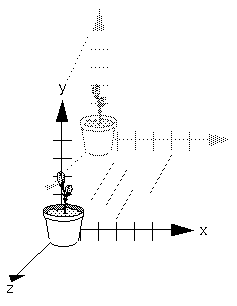Figure 3-5 : Translating an Object

Note that using (0.0, 0.0, 0.0) as the argument for glTranslate*() is the identity operation - that is, it has no effect on an object or its local coordinate system.

#### Rotate

void glRotate{fd}(TYPE angle, TYPE x, TYPE y, TYPE z);

Multiplies the current matrix by a matrix that rotates an object (or the local coordinate system) in a counterclockwise direction about the ray from the origin through the point (x, y, z). The angle parameter specifies the angle of rotation in degrees.

The effect of glRotatef(45.0, 0.0, 0.0, 1.0), which is a rotation of 45 degrees about the z-axis, is shown in Figure 3-6 .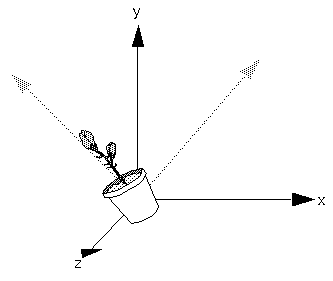Figure 3-6 : Rotating an Object

Note that an object that lies farther from the axis of rotation is more dramatically rotated (has a larger orbit) than an object drawn near the axis. Also, if the angle argument is zero, the glRotate*() command has no effect.

#### Scale

void glScale{fd}(TYPEx, TYPE y, TYPEz);

Multiplies the current matrix by a matrix that stretches, shrinks, or reflects an object along the axes. Each x, y, and z coordinate of every point in the object is multiplied by the corresponding argument x, y, or z. With the local coordinate system approach, the local coordinate axes are stretched by the x, y, and z factors, and the associated object is stretched with them.

Figure 3-7 shows the effect of glScalef(2.0, -0.5, 1.0).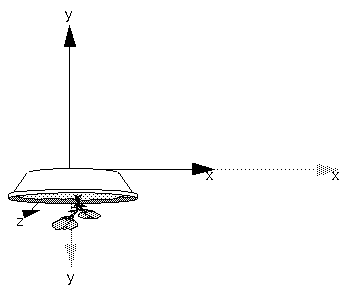Figure 3-7 : Scaling and Reflecting an Object

glScale*() is the only one of the three modeling transformations that changes the apparent size of an object: Scaling with values greater than 1.0 stretches an object, and using values less than 1.0 shrinks it. Scaling with a -1.0 value reflects an object across an axis. The identity values for scaling are (1.0, 1.0, 1.0). In general, you should limit your use of glScale*() to those cases where it is necessary. Using glScale*() decreases the performance of lighting calculations, because the normal vectors have to be renormalized after transformation.

A scale value of zero collapses all object coordinates along that axis to zero. It's usually not a good idea to do this, because such an operation cannot be undone. Mathematically speaking, the matrix cannot be inverted, and inverse matrices are required for certain lighting operations (see Chapter 6 ). Sometimes collapsing coordinates does make sense, however; the calculation of shadows on a planar surface is a typical application (see "Shadows," ). In general, if a coordinate system is to be collapsed, the projection matrix should be used rather than the modelview matrix.

#### A Modeling Transformation Code Example

Example 3-2 is a portion of a program that renders a triangle four times, as shown in Figure 3-8 :
A solid wireframe triangle is drawn with no modeling transformation.

The same triangle is drawn again, but with a dashed line stipple and translated.

A triangle is drawn with a long dashed line stipple, with its height (y-axis) halved and its width (x-axis) doubled.

A rotated, scaled triangle, made of dotted lines, is drawn.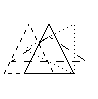Figure 3-8 : Modeling Transformation Example

Example 3-2 : Using Modeling Transformations: model.c

```glLoadIdentity();
glColor3f(1.0, 1.0, 1.0);
draw_triangle();                   /* solid lines */

glEnable(GL_LINE_STIPPLE);         /* dashed lines */
glLineStipple(1, 0xF0F0);
glTranslatef(-20.0, 0.0, 0.0);
draw_triangle();

glLineStipple(1, 0xF00F);          /*long dashed lines */
glScalef(1.5, 0.5, 1.0);
draw_triangle();

glLineStipple(1, 0x8888);          /* dotted lines */
glRotatef (90.0, 0.0, 0.0, 1.0);
draw_triangle ();
glDisable (GL_LINE_STIPPLE);```
Note the use of glLoadIdentity() to isolate the effects of modeling transformations; initializing the matrix values prevents successive transformations from having a cumulative effect. Even though using glLoadIdentity() repeatedly has the desired effect, it might be inefficient, depending on your particular OpenGL implementation. See "Manipulating the Matrix Stacks" for a better way to isolate transformations.

Sometimes, programmers who want a continuously rotating object attempt to achieve this by repeatedly applying a rotation matrix that has small values. The problem with this technique is that because of round-off errors, the product of thousands of tiny rotations gradually drifts away from the value you really want (it might even become something that isn't a rotation). Instead of using this technique, increment the angle and issue a new rotation command with the new angle at each update step.

### Viewing Transformations

A viewing transformation changes the position and orientation of the viewpoint. If you recall the camera analogy, the viewing transformation positions the camera tripod, pointing the camera toward the model. Just as you move the camera to some position and rotate it until it points in the desired direction, viewing transformations are generally composed of translations and rotations. Also remember that, to achieve a certain scene composition in the final image or photograph, either you can move the camera, or you can move all the objects in the opposite direction. Thus, a modeling transformation that rotates an object counterclockwise is equivalent to a viewing transformation that rotates the camera clockwise, for example. Finally, keep in mind that the viewing transformation commands must be called before any modeling transformations are performed, so that the modeling transformations take effect on the objects first.

You can accomplish a viewing transformation in any of several ways, as described below. You can also choose to use the default location and orientation of the viewpoint, which is at the origin, looking down the negative z-axis.

Use one or more modeling transformation commands (that is, glTranslate*() and glRotate*()). You can think of the effect of these transformations as moving the camera position or as moving all the objects in the world, relative to a stationary camera.

Use the Utility Library routine gluLookAt() to define a line of sight. This routine encapsulates a series of rotation and translation commands.

Create your own utility routine that encapsulates rotations and translations. Some applications might require custom routines that allow you to specify the viewing transformation in a convenient way. For example, you might want to specify the roll, pitch, and heading rotation angles of a plane in flight, or you might want to specify a transformation in terms of polar coordinates for a camera that's orbiting around an object.

#### Using glTranslate*() and glRotate*()

When you use modeling transformation commands to emulate viewing transformations, you're trying to move the viewpoint in a desired way while keeping the objects in the world stationary. Since the viewpoint is initially located at the origin and since objects are often most easily constructed there as well (see Figure 3-9 ), in general you have to perform some transformation so that the objects can be viewed. Note that, as shown in the figure, the camera initially points down the negative z-axis. (You're seeing the back of the camera.)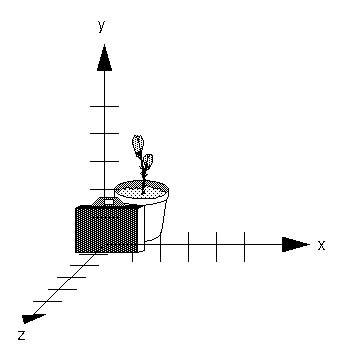Figure 3-9 : Object and Viewpoint at the Origin

In the simplest case, you can move the viewpoint backward, away from the objects; this has the same effect as moving the objects forward, or away from the viewpoint. Remember that by default forward is down the negative z-axis; if you rotate the viewpoint, forward has a different meaning. So, to put 5 units of distance between the viewpoint and the objects by moving the viewpoint, as shown in Figure 3-10 , use

`glTranslatef(0.0, 0.0, -5.0);`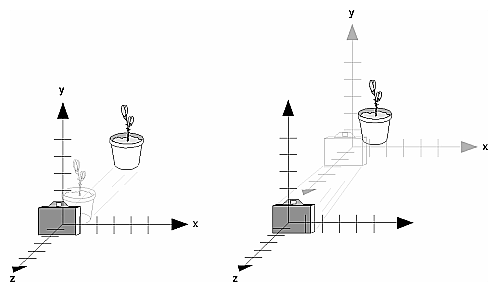Figure 3-10 : Separating the Viewpoint and the Object

Now suppose you want to view the objects from the side. Should you issue a rotate command before or after the translate command? If you're thinking in terms of a grand, fixed coordinate system, first imagine both the object and the camera at the origin. You could rotate the object first and then move it away from the camera so that the desired side is visible. Since you know that with the fixed coordinate system approach, commands have to be issued in the opposite order in which they should take effect, you know that you need to write the translate command first in your code and follow it with the rotate command.

Now let's use the local coordinate system approach. In this case, think about moving the object and its local coordinate system away from the origin; then, the rotate command is carried out using the now-translated coordinate system. With this approach, commands are issued in the order in which they're applied, so once again the translate command comes first. Thus, the sequence of transformation commands to produce the desired result is

```glTranslatef(0.0, 0.0, -5.0);
glRotatef(90.0, 0.0, 1.0, 0.0);```
If you're having trouble keeping track of the effect of successive matrix multiplications, try using both the fixed and local coordinate system approaches and see whether one makes more sense to you. Note that with the fixed coordinate system, rotations always occur about the grand origin, whereas with the local coordinate system, rotations occur about the origin of the local system. You might also try using the gluLookAt() utility routine described in the next section.

#### Using the gluLookAt() Utility Routine

Often, programmers construct a scene around the origin or some other convenient location, then they want to look at it from an arbitrary point to get a good view of it. As its name suggests, the gluLookAt() utility routine is designed for just this purpose. It takes three sets of arguments, which specify the location of the viewpoint, define a reference point toward which the camera is aimed, and indicate which direction is up. Choose the viewpoint to yield the desired view of the scene. The reference point is typically somewhere in the middle of the scene: If you've built your scene at the origin, the reference point is probably the origin. It might be a little trickier to specify the correct up-vector. Again, if you've built some real-world scene at or around the origin, and if you've been taking the positive y-axis to point upward, then that's your up-vector for gluLookAt(). However, if you're designing a flight simulator, up is the direction perpendicular to the plane's wings, from the plane toward the sky when the plane is right-side up on the ground.

The gluLookAt() routine is particularly useful when you want to pan across a landscape, for instance. With a viewing volume that's symmetric in both x and y, the (eyex, eyey, eyez) point specified is always in the center of the image on the screen, so you can use a series of commands to move this point slightly, thereby panning across the scene.void gluLookAt(GLdouble eyex, GLdouble eyey, GLdouble eyez, GLdouble centerx, GLdouble centery, GLdouble centerz, GLdouble upx, GLdouble upy, GLdouble upz);

Defines a viewing matrix and multiplies it to the right of the current matrix. The desired viewpoint is specified by eyex, eyey, and eyez. The centerx, centery, and centerz arguments specify any point along the desired line of sight, but typically they're some point in the center of the scene being looked at. The upx, upy, and upz arguments indicate which direction is up (that is, the direction from the bottom to the top of the viewing volume).

Note that gluLookAt() is part of the Utility Library rather than the basic OpenGL library. This isn't because it's not useful, but because it encapsulates several basic OpenGL commands - specifically, glTranslate*() and glRotate*(). To see this, imagine a camera located at an arbitrary viewpoint and oriented according to a line of sight, both as specified with gluLookAt(), and a scene located at the origin. To "undo" what gluLookAt() does, you need to transform the camera so that it sits at the origin and points down the negative z-axis, the default position. A simple translate moves the camera to the origin. You can easily imagine a series of rotations about each of the three axes of a fixed coordinate system that would orient the camera so that it pointed toward negative z values. Since OpenGL allows rotation about an arbitrary axis, you can accomplish any desired rotation of the camera with a single glRotate*() command.

To transform any arbitrary vector so that it's coincident with another arbitrary vector (for instance, the negative z-axis), you need to do a little mathematics. The axis about which you want to rotate is given by the cross product of the two normalized vectors. To find the angle of rotation, normalize the initial two vectors. The cosine of the desired angle between the vectors is equal to the dot product of the normalized vectors. To disambiguate between the two possible angles identified by the cosine (x degrees and x+180 degrees), recall that the length of the cross product of the normalized vectors equals the sine of the angle of rotation. (See Appendix F for definitions of cross and dot products.)

#### Creating a Custom Utility Routine

For some specialized applications, you might want to define your own transformation routine. Since this is rarely done and in any case is a fairly advanced topic, it's left mostly as an exercise for the reader. The following exercises suggest two custom viewing transformations that might be useful.

#### Try This:

Try This
Suppose you're writing a flight simulator and you'd like to display the world from the point of view of the pilot of a plane. The world is described in a coordinate system with the origin on the runway and the plane at coordinates (x, y, z). Suppose further that the plane has some roll, pitch, and heading (these are rotation angles of the plane relative to its center of gravity).

Show that the following routine could serve as the viewing transformation:

```void pilotView{GLdouble planex, GLdouble planey,
GLdouble planez, GLdouble roll,
{
glRotated(roll, 0.0, 0.0, 1.0);
glRotated(pitch, 0.0, 1.0, 0.0);
glTranslated(-planex, -planey, -planez);
}```
Suppose your application involves orbiting the camera around an object that's centered at the origin. In this case, you'd like to specify the viewing transformation by using polar coordinates. Let the distance variable define the radius of the orbit, or how far the camera is from the origin. (Initially, the camera is moved distance units along the positive z-axis.) The azimuth describes the angle of rotation of the camera about the object in the x-y plane, measured from the positive y-axis. Similarly, elevation is the angle of rotation of the camera in the y-z plane, measured from the positive z-axis. Finally, twist represents the rotation of the viewing volume around its line of sight.

Show that the following routine could serve as the viewing transformation:

```void polarView{GLdouble distance, GLdouble twist,
GLdouble elevation, GLdouble azimuth)
{
glTranslated(0.0, 0.0, -distance);
glRotated(-twist, 0.0, 0.0, 1.0);
glRotated(-elevation, 1.0, 0.0, 0.0);
glRotated(azimuth, 0.0, 0.0, 1.0);
}```

## Projection Transformations

The previous section described how to compose the desired modelview matrix so that the correct modeling and viewing transformations are applied. This section explains how to define the desired projection matrix, which is also used to transform the vertices in your scene. Before you issue any of the transformation commands described in this section, remember to call
```glMatrixMode(GL_PROJECTION);
so that the commands affect the projection matrix rather than the modelview matrix, and so that you avoid compound projection transformations. Since each projection transformation command completely describes a particular transformation, typically you don't want to combine a projection transformation with another transformation.

The purpose of the projection transformation is to define a viewing volume, which is used in two ways. The viewing volume determines how an object is projected onto the screen (that is, by using a perspective or an orthographic projection), and it defines which objects or portions of objects are clipped out of the final image. You can think of the viewpoint we've been talking about as existing at one end of the viewing volume. At this point, you might want to reread "A Simple Example: Drawing a Cube," for its overview of all the transformations, including projection transformations.

### Perspective Projection

The most unmistakable characteristic of perspective projection is foreshortening: the farther an object is from the camera, the smaller it appears in the final image. This occurs because the viewing volume for a perspective projection is a frustum of a pyramid (a truncated pyramid whose top has been cut off by a plane parallel to its base). Objects that fall within the viewing volume are projected toward the apex of the pyramid, where the camera or viewpoint is. Objects that are closer to the viewpoint appear larger because they occupy a proportionally larger amount of the viewing volume than those that are farther away, in the larger part of the frustum. This method of projection is commonly used for animation, visual simulation, and any other applications that strive for some degree of realism because it's similar to how our eye (or a camera) works.

The command to define a frustum, glFrustum(), calculates a matrix that accomplishes perspective projection and multiplies the current projection matrix (typically the identity matrix) by it. Recall that the viewing volume is used to clip objects that lie outside of it; the four sides of the frustum, its top, and its base correspond to the six clipping planes of the viewing volume, as shown in Figure 3-11 . Objects or parts of objects outside these planes are clipped from the final image. Note that glFrustum() doesn't require you to define a symmetric viewing volume.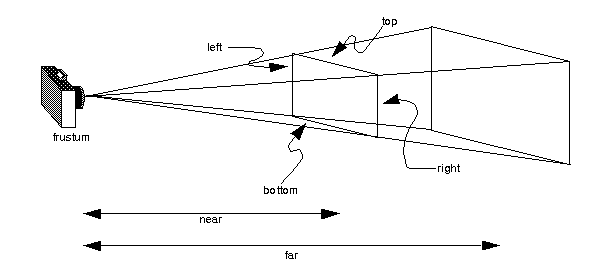Figure 3-11 : The Perspective Viewing Volume Specified by glFrustum()

void glFrustum(GLdouble left, GLdouble right, GLdouble bottom, GLdouble top, GLdouble near, GLdouble far);

Creates a matrix for a perspective-view frustum and multiplies the current matrix by it. The frustum's viewing volume is defined by the parameters: (left, bottom, -near) and (right, top, -near) specify the (x, y, z) coordinates of the lower left and upper right corners of the near clipping plane; near and far give the distances from the viewpoint to the near and far clipping planes. They should always be positive.

The frustum has a default orientation in three-dimensional space. You can perform rotations or translations on the projection matrix to alter this orientation, but this is tricky and nearly always avoidable.

Also, the frustum doesn't have to be symmetrical, and its axis isn't necessarily aligned with the z-axis. For example, you can use glFrustum() to draw a picture as if you were looking through a rectangular window of a house, where the window was above and to the right of you. Photographers use such a viewing volume to create false perspectives. You might use it to have the hardware calculate images at much higher than normal resolutions, perhaps for use on a printer. For example, if you want an image that has twice the resolution of your screen, draw the same picture four times, each time using the frustum to cover the entire screen with one-quarter of the image. After each quarter of the image is rendered, you can read the pixels back to collect the data for the higher-resolution image. (See Chapter 8 for more information about reading pixel data.)

Although it's easy to understand conceptually, glFrustum() isn't intuitive to use. Instead, you might try the Utility Library routine gluPerspective(). This routine creates a viewing volume of the same shape as glFrustum() does, but you specify it in a different way. Rather than specifying corners of the near clipping plane, you specify the angle of the field of view in the x-z plane and the aspect ratio of the width to height (x/y). (For a square portion of the screen, the aspect ratio is 1.0.) These two parameters are enough to determine an untruncated pyramid along the line of sight, as shown in Figure 3-12 . You also specify the distance between the viewpoint and the near and far clipping planes, thereby truncating the pyramid. Note that gluPerspective() is limited to creating frustums that are symmetric in both the x- and y-axes along the line of sight, but this is usually what you want.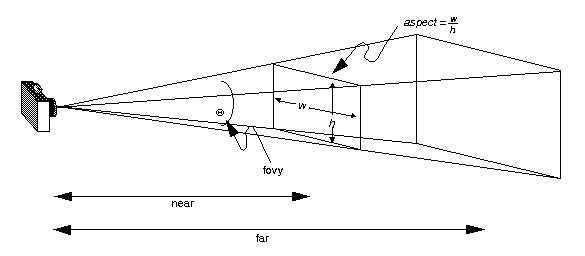Figure 3-12 : The Perspective Viewing Volume Specified by gluPerspective()

void gluPerspective(GLdouble fovy, GLdouble aspect, GLdouble zNear, GLdouble zFar);

Creates a matrix for a symmetric perspective-view frustum and multiplies the current matrix by it. The fovy argument is the angle of the field of view in the x-z plane; its value must be in the range [0.0,180.0]. The aspect ratio is the width of the frustum divided by its height. The zNear and zFar values are the distances between the viewpoint and the clipping planes, along the negative z-axis. They should always be positive.

Just as with glFrustum(), you can apply rotations or translations to change the default orientation of the viewing volume created by gluPerspective(). With no such transformations, the viewpoint remains at the origin, and the line of sight points down the negative z-axis.

With gluPerspective(), you need to pick appropriate values for the field of view, or the image may look distorted. For example, suppose you're drawing to the entire screen, which happens to be 11 inches high. If you choose a field of view of 90 degrees, your eye has to be about 7.8 inches from the screen for the image to appear undistorted. (This is the distance that makes the screen subtend 90 degrees.) If your eye is farther from the screen, as it usually is, the perspective doesn't look right. If your drawing area occupies less than the full screen, your eye has to be even closer. To get a perfect field of view, figure out how far your eye normally is from the screen and how big the window is, and calculate the angle the window subtends at that size and distance. It's probably smaller than you would guess. Another way to think about it is that a 94-degree field of view with a 35-millimeter camera requires a 20-millimeter lens, which is a very wide-angle lens. "Troubleshooting Transformations," gives more details on how to calculate the desired field of view.

The preceding paragraph mentions inches and millimeters - do these really have anything to do with OpenGL? The answer is, in a word, no. The projection and other transformations are inherently unitless. If you want to think of the near and far clipping planes as located at 1.0 and 20.0 meters, inches, kilometers, or leagues, it's up to you. The only rule is that you have to use a consistent unit of measurement. Then the resulting image is drawn to scale.

### Orthographic Projection

With an orthographic projection, the viewing volume is a rectangular parallelepiped, or more informally, a box (see Figure 3-13 ). Unlike perspective projection, the size of the viewing volume doesn't change from one end to the other, so distance from the camera doesn't affect how large an object appears. This type of projection is used for applications such as creating architectural blueprints and computer-aided design, where it's crucial to maintain the actual sizes of objects and angles between them as they're projected.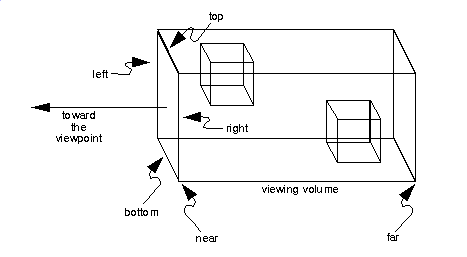Figure 3-13 : The Orthographic Viewing Volume

The command glOrtho() creates an orthographic parallel viewing volume. As with glFrustum(), you specify the corners of the near clipping plane and the distance to the far clipping plane. void glOrtho(GLdouble left, GLdouble right, GLdouble bottom,
GLdouble top, GLdouble near, GLdouble far);

Creates a matrix for an orthographic parallel viewing volume and multiplies the current matrix by it. The near clipping plane is a rectangle with the lower left corner at (left, bottom, -near) and the upper right corner at (right, top, -near). The far clipping plane is a rectangle with corners at (left, bottom, -far) and (right, top, -far). Both near and far can be positive or negative.

With no other transformations, the direction of projection is parallel to the z-axis, and the viewpoint faces toward the negative z-axis. Note that this means that the values passed in for far and near are used as negative z values if these planes are in front of the viewpoint, and positive if they're behind the viewpoint.

For the special case of projecting a two-dimensional image onto a two-dimensional screen, use the Utility Library routine gluOrtho2D(). This routine is identical to the three-dimensional version, glOrtho(), except that all the z coordinates for objects in the scene are assumed to lie between -1.0 and 1.0. If you're drawing two-dimensional objects using the two-dimensional vertex commands, all the z coordinates are zero; thus, none of the objects are clipped because of their z values. void gluOrtho2D(GLdouble left, GLdouble right, GLdouble bottom, GLdouble top);

Creates a matrix for projecting two-dimensional coordinates onto the screen and multiplies the current projection matrix by it. The clipping plane is a rectangle with the lower left corner at (left, bottom) and the upper right corner at (right, top).

### Viewing Volume Clipping

After the vertices of the objects in the scene have been transformed by the modelview and projection matrices, any vertices that lie outside the viewing volume are clipped. The six clipping planes used are those that define the sides and ends of the viewing volume. You can specify additional clipping planes and locate them wherever you choose; this relatively advanced topic is discussed in "Additional Clipping Planes." Keep in mind that OpenGL reconstructs the edges of polygons that get clipped.

## Viewport Transformation

Recalling the camera analogy, the viewport transformation corresponds to the stage where the size of the developed photograph is chosen. Do you want a wallet-size or a poster-size photograph? Since this is computer graphics, the viewport is the rectangular region of the window where the image is drawn. Figure 3-14 shows a viewport that occupies most of the screen. The viewport is measured in window coordinates, which reflect the position of pixels on the screen relative to the lower left corner of the window. Keep in mind that all vertices have been transformed by the modelview and projection matrices by this point, and vertices outside the viewing volume have been clipped.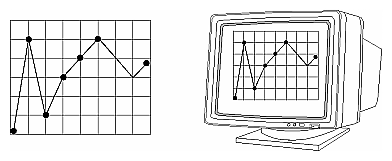Figure 3-14 : A Viewport Rectangle

### Defining the Viewport

The window manager, not OpenGL, is responsible for opening a window on the screen. However, by default the viewport is set to the entire pixel rectangle of the window that's opened. You use the glViewport() command to choose a smaller drawing region; for example, you can subdivide the window to create a split-screen effect for multiple views in the same window. void glViewport(GLint x, GLint y, GLsizei width, GLsizei height);

Defines a pixel rectangle in the window into which the final image is mapped. The (x, y) parameter specifies the lower left corner of the viewport, and width and height are the size of the viewport rectangle. By default, the initial viewport values are (0, 0, winWidth, winHeight), where winWidth and winHeight are the size of the window.

The aspect ratio of a viewport should generally equal the aspect ratio of the viewing volume. If the two ratios are different, the projected image will be distorted as it's mapped to the viewport, as shown in Figure 3-15 . Note that subsequent changes to the size of the window don't explicitly affect the viewport. Your application should detect window resize events and modify the viewport appropriately.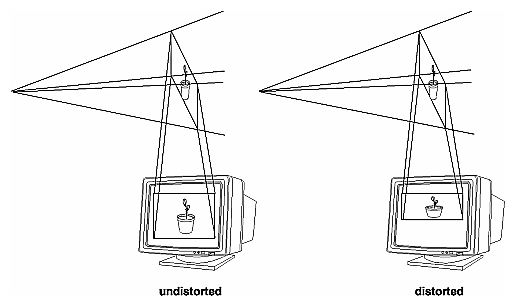Figure 3-15 : Mapping the Viewing Volume to the Viewport

For example, this sequence maps a square image onto a square viewport:

```gluPerspective(myFovy, 1.0, myNear, myFar);
glViewport(0, 0, 400, 400);```
However, the following sequence projects a nonequilateral rectangular image onto a square viewport. The image appears compressed along the x-axis, as shown in Figure 3-15 .
```gluPerspective(myFovy, 2.0, myNear, myFar);
glViewport (0, 0, 400, 400);```
To avoid the distortion, this line could be used:
`glViewport(0, 0, 400, 200);`

#### Try This

Try This
Modify an existing program so that an object is drawn twice, in different viewports. You might draw the object with different projection and/or viewing transformations for each viewport. To create two side-by-side viewports, you might issue these commands, along with the appropriate modeling, viewing, and projection transformations:
```glViewport (0, 0, sizex/2, sizey);
.
.
.
glViewport (sizex/2, 0, sizex/2, sizey);```

### The Transformed z Coordinate

The z or depth coordinate is encoded and then stored during the viewport transformation. You can scale z values to lie within a desired range with the glDepthRange() command. (Chapter 10 discusses the depth buffer and the corresponding uses for the z coordinate.) Unlike x and y window coordinates, z window coordinates are treated by OpenGL as though they always range from 0.0 to 1.0. void glDepthRange(GLclampd near, GLclampd far);

Defines an encoding for z coordinates that's performed during the viewport transformation. The near and far values represent adjustments to the minimum and maximum values that can be stored in the depth buffer. By default, they're 0.0 and 1.0, respectively, which work for most applications. These parameters are clamped to lie within [0,1].

## Troubleshooting Transformations

It's pretty easy to get a camera pointed in the right direction, but in computer graphics, you have to specify position and direction with coordinates and angles. As we can attest, it's all too easy to achieve the well-known black-screen effect. Although any number of things can go wrong, often you get this effect - which results in absolutely nothing being drawn in the window you open on the screen - from incorrectly aiming the "camera" and taking a picture with the model behind you. A similar problem arises if you don't choose a field of view that's wide enough to view your objects but narrow enough so they appear reasonably large.

If you find yourself exerting great programming effort only to create a black window, try these diagnostic steps:

Check the obvious possibilities. Make sure your system is plugged in. Make sure you're drawing your objects with a color that's different from the color with which you're clearing the screen. Make sure that whatever states you're using (such as lighting, texturing, alpha blending, logical operations, or antialiasing) are correctly turned on or off, as desired.

Remember that with the projection commands, the near and far coordinates measure distance from the viewpoint and that (by default) you're looking down the negative z axis. Thus, if the near value is 1.0 and the far 3.0, objects must have z coordinates between -1.0 and -3.0 in order to be visible. To ensure that you haven't clipped everything out of your scene, temporarily set the near and far clipping planes to some absurdly inclusive values, such as 0.001 and 1000000.0. This might negatively affect performance for such operations as depth-buffering and fog, but it might uncover inadvertently clipped objects.

Determine where the viewpoint is, in which direction you're looking, and where your objects are. It might help to create a real three-dimensional space - using your hands, for instance - to figure these things out.

Make sure you know where you're rotating about. You might be rotating about some arbitrary location unless you translated back to the origin first. It's OK to rotate about any point unless you're expecting to rotate about the origin.

Check your aim. Use gluLookAt() to aim the viewing volume at your objects. Or draw your objects at or near the origin, and use glTranslate*() as a viewing transformation to move the camera far enough in the z direction only, so that the objects fall within the viewing volume. Once you've managed to make your objects visible, try to incrementally change the viewing volume to achieve the exact result you want, as described below.

Even after you've aimed the camera in the correct direction and you can see your objects, they might appear too small or too large. If you're using gluPerspective(), you might need to alter the angle defining the field of view by changing the value of the first parameter for this command. You can use trigonometry to calculate the desired field of view given the size of the object and its distance from the viewpoint: The tangent of half the desired angle is half the size of the object divided by the distance to the object (see Figure 3-16 ). Thus, you can use an arctangent routine to compute half the desired angle. Example 3-3 assumes such a routine, atan2(), which calculates the arctangent given the length of the opposite and adjacent sides of a right triangle. This result then needs to be converted from radians to degrees.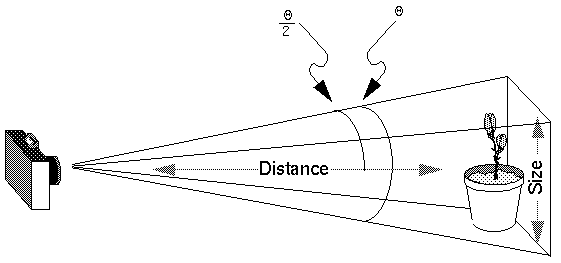Figure 3-16 : Using Trigonometry to Calculate the Field of View

Example 3-3 : Calculating Field of View

```#define PI 3.1415926535

double calculateAngle(double size, double distance)
{

radtheta = 2.0 * atan2 (size/2.0, distance);
degtheta = (180.0 * radtheta) / PI;
return (degtheta);
}```
Of course, typically you don't know the exact size of an object, and the distance can only be determined between the viewpoint and a single point in your scene. To obtain a fairly good approximate value, find the bounding box for your scene by determining the maximum and minimum x, y, and z coordinates of all the objects in your scene. Then calculate the radius of a bounding sphere for that box, and use the center of the sphere to determine the distance and the radius to determine the size.

For example, suppose all the coordinates in your object satisfy the equations -1 &le; x &le; 3, 5 &le; y &le; 7, and -5 &le; z &le; 5. Then, the center of the bounding box is (1, 6, 0), and the radius of a bounding sphere is the distance from the center of the box to any corner - say (3, 7, 5) - or: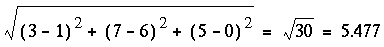If the viewpoint is at (8, 9, 10), the distance between it and the center is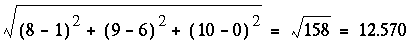The tangent of the half angle is 5.477 divided by 12.570, or 0.4357, so the half angle is 23.54 degrees.

Remember that the field-of-view angle affects the optimal position for the viewpoint, if you're trying to achieve a realistic image. For example, if your calculations indicate that you need a 179-degree field of view, the viewpoint must be a fraction of an inch from the screen to achieve realism. If your calculated field of view is too large, you might need to move the viewpoint farther away from the object.

## Manipulating the Matrix Stacks

The modelview and projection matrices you've been creating, loading, and multiplying have only been the visible tips of their respective icebergs: Each of these matrices is actually the topmost member of a stack of matrices (see Figure 3-17 ).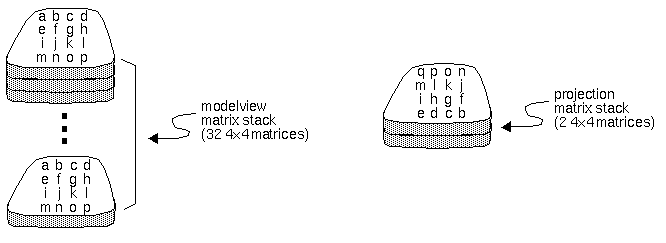Figure 3-17 : Modelview and Projection Matrix Stacks

A stack of matrices is useful for constructing hierarchical models, in which complicated objects are constructed from simpler ones. For example, suppose you're drawing an automobile that has four wheels, each of which is attached to the car with five bolts. You have a single routine to draw a wheel and another to draw a bolt, since all the wheels and all the bolts look the same. These routines draw a wheel or a bolt in some convenient position and orientation, say centered at the origin with its axis coincident with the z axis. When you draw the car, including the wheels and bolts, you want to call the wheel-drawing routine four times with different transformations in effect each time to position the wheels correctly. As you draw each wheel, you want to draw the bolts five times, each time translated appropriately relative to the wheel.

Suppose for a minute that all you have to do is draw the car body and the wheels. The English description of what you want to do might be something like this:

Draw the car body. Remember where you are, and translate to the right front wheel. Draw the wheel and throw away the last translation so your current position is back at the origin of the car body. Remember where you are, and translate to the left front wheel....

Similarly, for each wheel, you want to draw the wheel, remember where you are, and successively translate to each of the positions that bolts are drawn, throwing away the transformations after each bolt is drawn.

Since the transformations are stored as matrices, a matrix stack provides an ideal mechanism for doing this sort of successive remembering, translating, and throwing away. All the matrix operations that have been described so far (glLoadMatrix(), glMultMatrix(), glLoadIdentity(), and the commands that create specific transformation matrices) deal with the current matrix, or the top matrix on the stack. You can control which matrix is on top with the commands that perform stack operations: glPushMatrix(), which copies the current matrix and adds the copy to the top of the stack, and glPopMatrix(), which discards the top matrix on the stack, as shown in Figure 3-18 . (Remember that the current matrix is always the matrix on the top.) In effect, glPushMatrix() means "remember where you are" and glPopMatrix() means "go back to where you were."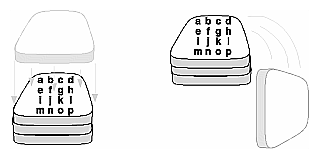Figure 3-18 : Pushing and Popping the Matrix Stack

void glPushMatrix(void);

Pushes all matrices in the current stack down one level. The current stack is determined by glMatrixMode(). The topmost matrix is copied, so its contents are duplicated in both the top and second-from-the-top matrix. If too many matrices are pushed, an error is generated.

void glPopMatrix(void);

Pops the top matrix off the stack. What was the second-from-the-top matrix becomes the top matrix. The current stack is determined by glMatrixMode(). The contents of the topmost matrix are destroyed. If the stack contains a single matrix, calling glPopMatrix() generates an error.

Example 3-4 draws an automobile, assuming the existence of routines that draw the car body, a wheel, and a bolt.

Example 3-4 : Pushing and Popping the Matrix

```draw_wheel_and_bolts()
{
long i;

draw_wheel();
for(i=0;i<5;i++){
glPushMatrix();
glRotatef(72.0*i,0.0,0.0,1.0);
glTranslatef(3.0,0.0,0.0);
draw_bolt();
glPopMatrix();
}
}

draw_body_and_wheel_and_bolts()
{
draw_car_body();
glPushMatrix();
glTranslatef(40,0,30);                     /*move to first wheel position*/
draw_wheel_and_bolts();
glPopMatrix();
glPushMatrix();
glTranslatef(40,0,-30);                     /*move to 2nd wheel position*/
draw_wheel_and_bolts();
glPopMatrix();
...                  /*draw last two wheels similarly*/
}```
This code assumes the wheel and bolt axes are coincident with the z-axis, that the bolts are evenly spaced every 72 degrees, 3 units (maybe inches) from the center of the wheel, and that the front wheels are 40 units in front of and 30 units to the right and left of the car's origin.

A stack is more efficient than an individual matrix, especially if the stack is implemented in hardware. When you push a matrix, you don't need to copy the current data back to the main process, and the hardware may be able to copy more than one element of the matrix at a time. Sometimes you might want to keep an identity matrix at the bottom of the stack so that you don't need to call glLoadIdentity() repeatedly.

### The Modelview Matrix Stack

As you've seen earlier in this chapter, the modelview matrix contains the cumulative product of multiplying viewing and modeling transformation matrices. Each viewing or modeling transformation creates a new matrix that multiplies the current modelview matrix; the result, which becomes the new current matrix, represents the composite transformation. The modelview matrix stack contains at least thirty-two 4 × 4 matrices; initially, the topmost matrix is the identity matrix. Some implementations of OpenGL may support more than thirty-two matrices on the stack. You can use the query command glGetIntegerv() with the argument GL_MAX_MODELVIEW_STACK_DEPTH to find the maximum allowable number of matrices.

### The Projection Matrix Stack

The projection matrix contains a matrix for the projection transformation, which describes the viewing volume. Generally, you don't want to compose projection matrices, so you issue glLoadIdentity() before performing a projection transformation. Also for this reason, the projection matrix stack need be only two levels deep; some OpenGL implementations may allow more than two 4 × 4 matrices. (You can use glGetIntegerv() with GL_MAX_PROJECTION_STACK_DEPTH as the argument to find the stack depth.)

One use for a second matrix in the stack would be an application that needs to display a help window with text in it, in addition to its normal window showing a three-dimensional scene. Since text is most easily drawn with an orthographic projection, you could change temporarily to an orthographic projection, display the help, and then return to your previous projection:

```glMatrixMode(GL_PROJECTION);
glPushMatrix();                        /*save the current projection*/
glOrtho(...);                        /*set up for displaying help*/
display_the_help();
glPopMatrix();```
Note that you'd probably have to also change the modelview matrix appropriately.

If you know enough mathematics, you can create custom projection matrices that perform arbitrary projective transformations. For example, the OpenGL and its Utility Library have no built-in mechanism for two-point perspective. If you were trying to emulate the drawings in drafting texts, you might need such a projection matrix.

In addition to the six clipping planes of the viewing volume (left, right, bottom, top, near, and far), you can define up to six additional clipping planes to further restrict the viewing volume, as shown in Figure 3-19 . This is useful for removing extraneous objects in a scene - for example, if you want to display a cutaway view of an object.

Each plane is specified by the coefficients of its equation: Ax+By+Cz+D = 0. The clipping planes are automatically transformed appropriately by modeling and viewing transformations. The clipping volume becomes the intersection of the viewing volume and all half-spaces defined by the additional clipping planes. Remember that polygons that get clipped automatically have their edges reconstructed appropriately by OpenGL.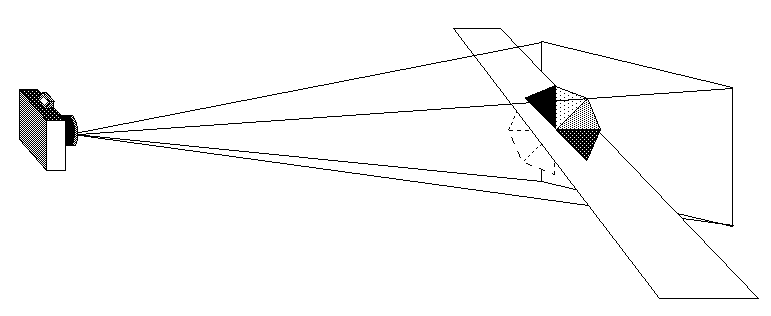Figure 3-19 : Additional Clipping Planes and the Viewing Volume

void glClipPlane(GLenum plane, const GLdouble *equation);

Defines a clipping plane. The equation argument points to the four coefficients of the plane equation, Ax+By+Cz+D = 0. All points with eye coordinates (xe, ye, ze, we) that satisfy (A B C D)M-1 (xe ye ze we)T >= 0 lie in the half-space defined by the plane, where M is the current modelview matrix at the time glClipPlane() is called. All points not in this half-space are clipped away. The plane argument is GL_CLIP_PLANEi, where i is an integer between 0 and 5, specifying which of the six clipping planes to define.

Clipping performed as a result of glClipPlane() is done in eye coordinates, not in clip coordinates. This difference is noticeable if the projection matrix is singular (that is, a real projection matrix that flattens three-dimensional coordinates to two-dimensional ones). Clipping performed in eye coordinates continues to take place in three dimensions even when the projection matrix is singular.

You need to enable each additional clipping plane you define:

`glEnable(GL_CLIP_PLANEi);`
You can disable a plane with
`glDisable(GL_CLIP_PLANEi);`
Some implementations may allow more than six clipping planes. You can use glGetIntegerv() with GL_MAX_CLIP_PLANES to find how many clipping planes are supported.

#### A Clipping Plane Code Example

Example 3-5 renders a wireframe sphere with two clipping planes that slice away three-quarters of the original sphere, as shown in Figure 3-20 .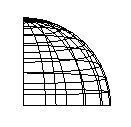Figure 3-20 : A Clipped Wireframe Sphere

Example 3-5 : A Wireframe Sphere with Two Clipping Planes: clip.c

```#include <GL/gl.h>
#include <GL/glu.h>
#include "aux.h"

void display(void)
{
GLdouble eqn = {0.0, 1.0, 0.0, 0.0};    /* y < 0 */
GLdouble eqn2 = {1.0, 0.0, 0.0, 0.0};   /* x < 0 */

glClear(GL_COLOR_BUFFER_BIT);

glColor3f (1.0, 1.0, 1.0);
glPushMatrix();
glTranslatef (0.0, 0.0, -5.0);

glClipPlane (GL_CLIP_PLANE0, eqn);
glEnable (GL_CLIP_PLANE0);
glClipPlane (GL_CLIP_PLANE1, eqn2);
glEnable (GL_CLIP_PLANE1);

glRotatef (90.0, 1.0, 0.0, 0.0);
auxWireSphere(1.0);
glPopMatrix();
glFlush();
}

void myinit (void)
{
}

void myReshape(GLsizei w, GLsizei h)
{
glViewport(0, 0, w, h);
glMatrixMode(GL_PROJECTION);
gluPerspective(60.0, (GLfloat) w/(GLfloat) h, 1.0, 20.0);
glMatrixMode(GL_MODELVIEW);
}

int main(int argc, char** argv)
{
auxInitDisplayMode (AUX_SINGLE | AUX_RGBA);
auxInitPosition (0, 0, 500, 500);
auxInitWindow (argv);
myinit ();
auxReshapeFunc (myReshape);
auxMainLoop(display);
}```

#### Try This

Try This
Try changing the coefficients that describe the clipping planes in Example 3-5 .

Try calling a modeling transformation, such as glRotate*(), to affect glClipPlane(). Make the clipping plane move independently of the objects in the scene.

## Examples of Composing Several Transformations

This section demonstrates how to combine several transformations to achieve a particular result. The two examples discussed are a solar system, in which objects need to rotate on their axes as well as in orbit around each other, and a robot arm, which has several joints that effectively transform coordinate systems as they move relative to each other.
Building a Solar System
Building an Articulated Robot Arm

### Building a Solar System

The program described in this section draws a simple solar system with a planet and a sun, both using the same sphere-drawing routine. To write this program, you need to use glRotate*() for the revolution of the planet around the sun and for the rotation of the planet around its own axis. You also need glTranslate*() to move the planet out to its orbit, away from the origin of the solar system. Remember that you can specify the desired size of the two spheres by supplying the appropriate arguments for the auxSphere() routine.

To draw the solar system, you first want to set up a projection and a viewing transformation. For this example, gluPerspective() and gluLookAt() are used.

Drawing the sun is straightforward, since it should be located at the origin of the grand, fixed coordinate system, which is where the sphere routine places it. Thus, drawing the sun doesn't require translation; you can use glRotate*() to make the sun rotate about an arbitrary axis. To draw a planet rotating around the sun, as shown in Figure 3-21 , requires several modeling transformations. The planet needs to rotate about its own axis once a day. And once a year, the planet completes one revolution around the sun.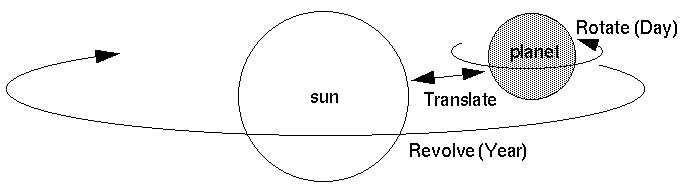Figure 3-21 : Planet and Sun

To determine the order of modeling transformations, visualize what happens to the local coordinate system. An initial glRotate*() rotates the local coordinate system that initially coincides with the grand coordinate system. Next, glTranslate*() moves the local coordinate system to a position on the planet's orbit; the distance moved should equal the radius of the orbit. Thus, the initial glRotate*() actually determines where along the orbit the planet is (or what time of year it is).

A second glRotate*() rotates the local coordinate system around the local axes, thus determining the time of day for the planet. Once you've issued all these transformation commands, the planet can be drawn.

In summary, these are the OpenGL commands to draw the sun and planet; the full program is shown in Example 3-6 .

```glPushMatrix();
auxWireSphere(1.0);                /* draw sun */
glRotatef ((GLfloat) year, 0.0, 1.0, 0.0);
glTranslatef (2.0, 0.0, 0.0);
glRotatef ((GLfloat) day, 0.0, 1.0, 0.0);
auxWireSphere(0.2);               /* draw smaller planet */
glPopMatrix();```
Example 3-6 : A Planetary System: planet.c
```#include <GL/gl.h>
#include <GL/glu.h>
#include "aux.h"

static int year = 0, day = 0;

{
day = (day + 10) % 360;
}

void daySubtract (void)
{
day = (day - 10) % 360;
}

{
year = (year + 5) % 360;
}

void yearSubtract (void)
{
year = (year - 5) % 360;
}

void display(void)
{
glClear(GL_COLOR_BUFFER_BIT);

glColor3f (1.0, 1.0, 1.0);
glPushMatrix();
auxWireSphere(1.0);        /* draw sun */
glRotatef((GLfloat) year, 0.0, 1.0, 0.0);
glTranslatef (2.0, 0.0, 0.0);
glRotatef((GLfloat) day, 0.0, 1.0, 0.0);
auxWireSphere(0.2);        /* draw smaller planet */
glPopMatrix();
glFlush();
}

void myinit(void)
{
}

void myReshape(GLsizei w, GLsizei h)
{
glViewport(0, 0, w, h);
glMatrixMode(GL_PROJECTION);
gluPerspective(60.0, (GLfloat) w/(GLfloat) h, 1.0, 20.0);
glMatrixMode(GL_MODELVIEW);
glTranslatef (0.0, 0.0, -5.0);
}

int main(int argc, char** argv)
{
auxInitDisplayMode(AUX_SINGLE | AUX_RGBA);
auxInitPosition(0, 0, 500, 500);
auxInitWindow(argv);
myinit();
auxKeyFunc(AUX_LEFT, yearSubtract);
auxKeyFunc(AUX_DOWN, daySubtract);
auxReshapeFunc(myReshape);
auxMainLoop(display);
}```

#### Try This

Try This
Try adding a moon to the planet. Or try several moons and additional planets. Hint: Use glPushMatrix() and glPopMatrix() to save and restore the position and orientation of the coordinate system at appropriate moments. If you're going to draw several moons around a planet, you need to save the coordinate system prior to positioning each moon and restore the coordinate system after each moon is drawn.

Try tilting the planet's axis.

### Building an Articulated Robot Arm

This section discusses a program that creates an articulated robot arm with two or more segments. The arm should be connected with pivot points at the shoulder, elbow, or other joints. Figure 3-22 shows a single joint of such an arm.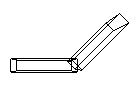Figure 3-22 : A Robot Arm

You can use a scaled box as a segment of the robot arm, but first you must call the appropriate modeling transformations to orient each segment. Since the origin of the local coordinate system is initially at the center of the box, you need to move the local coordinate system to one edge of the box. Otherwise, the box rotates about its center rather than the pivot point.

After you call glTranslate*() to establish the pivot point and glRotate*() to pivot the box, translate back to the center of the box and then draw the box. Here's what your code might look like for this first segment of the arm (the entire program is shown in Example 3-7 ):

```glTranslatef (-1.0, 0.0, 0.0);
glRotatef ((GLfloat) shoulder_angle, 0.0, 0.0, 1.0);
glTranslatef (1.0, 0.0, 0.0);
auxWireBox(2.0, 0.4, 1.0);```
To build a second segment, you need to move the local coordinate system to the next pivot point. Since the coordinate system has previously been rotated, the x-axis is already oriented along the length of the rotated arm. Therefore, translating along the x-axis moves the local coordinate system to the next pivot point. Once it's at that pivot point, you can use the same code to draw the second segment as you used for the first one. This can be continued for an indefinite number of segments (shoulder, elbow, wrist, fingers).
```glTranslatef (1.0, 0.0, 0.0);
glRotatef ((GLfloat) elbow_angle, 0.0, 0.0, 1.0);
glTranslatef (1.0, 0.0, 0.0);
auxWireBox(2.0, 0.4, 1.0);```
Example 3-7 : A Robot Arm: robot.c
```#include <GL/gl.h>
#include <GL/glu.h>
#include "aux.h"

static int shoulder = 0, elbow = 0;

{
elbow = (elbow + 5) % 360;
}

void elbowSubtract (void)
{
elbow = (elbow - 5) % 360;
}

{
shoulder = (shoulder + 5) % 360;
}

void shoulderSubtract (void)
{
shoulder = (shoulder - 5) % 360;
}

void display(void)
{
glClear(GL_COLOR_BUFFER_BIT);
glColor3f (1.0, 1.0, 1.0);

glPushMatrix();
glTranslatef (-1.0, 0.0, 0.0);
glRotatef ((GLfloat) shoulder, 0.0, 0.0, 1.0);
glTranslatef (1.0, 0.0, 0.0);
auxWireBox(2.0, 0.4, 1.0);      /* shoulder */

glTranslatef (1.0, 0.0, 0.0);
glRotatef ((GLfloat) elbow, 0.0, 0.0, 1.0);
glTranslatef (1.0, 0.0, 0.0);
auxWireBox(2.0, 0.4, 1.0);      /* elbow */

glPopMatrix();
glFlush();
}

void myinit (void)
{
}

void myReshape(GLsizei w, GLsizei h)
{
glViewport(0, 0, w, h);
glMatrixMode(GL_PROJECTION);
gluPerspective(65.0, (GLfloat) w/(GLfloat) h, 1.0, 20.0);
glMatrixMode(GL_MODELVIEW);
glTranslatef(0.0, 0.0, -5.0);
}

int main(int argc, char** argv)
{
auxInitDisplayMode (AUX_SINGLE | AUX_RGBA);
auxInitPosition (0, 0, 400, 400);
auxInitWindow (argv);
myinit ();
auxKeyFunc (AUX_LEFT, shoulderSubtract);
auxKeyFunc (AUX_DOWN, elbowSubtract);
auxReshapeFunc (myReshape);
auxMainLoop(display);
}```

#### Try This

Try This